Section D

# Improved Single Feistel Circuit Supporter by A Chaotic Genetic Operator

Abdellatif JarJar 1 , *
1High School Moulay Rachid, Taza, Morroco, abdoujjar@gmail.com
*Corresponding Author: High School Moulay Rachid, Taza, Morroco, abdoujjar@gmail.com.

© Copyright 2020 Korea Multimedia Society. This is an Open-Access article distributed under the terms of the Creative Commons Attribution Non-Commercial License (http://creativecommons.org/licenses/by-nc/4.0/) which permits unrestricted non-commercial use, distribution, and reproduction in any medium, provided the original work is properly cited.

Received: Apr 11, 2020; Revised: Apr 23, 2020; Accepted: May 16, 2020

Published Online: Jun 30, 2020

## Abstract

This document outlines a new color image encryption technology development. After splitting the original image into 240-bit blocks and modifying the first block by an initialization vector, an improved Feistel circuit is applied, sponsored by a genetic crossover operator and then strong chaining between the encrypted block and the next clear block is attached to set up the confusion-diffusion and heighten the avalanche effect, which protects the system from any known attack. Simulations carried out on a large database of color images of different sizes and formats prove the robustness of such a system.

Keywords: Chaotic map; Feistel-round diagram; genetic operator; S-Box; P-Box

## I. INTRODUCTION

With the rapid advance of innovative technologies in the field of information science and the digital world, all data are increasingly shared over Internet networks. On the other hand, unauthorized access to information or private information has become a problem in the virtual world. Security issues are increasingly coming to the forefront. Encryption and tattooing have become the most effective way to protect against unexpected attacks. However, traditional encryption standards, such as DES and AES, are generally designed only for encrypting text that does not have a high correlation, and are therefore considered unsuitable for images and video data sequences. The new vision of image-based encryption is the use of chaotic sequences for encryption key generation first prescribed by Friedrich in 1998. Since the image has been introduced and processed in digital form, its applications have been steadily increasing. It is now exploited by a wide public, both professional and amateur. However, given the extent of computer resources allowing the free circulation of information and the ease of transmission of confidential data, man has been pushed to increasingly improve encryption algorithms to secure his confidential data. To protect against known attacks, any new encryption system must agree to Shannon’s recommendations ; (Permutation, confusion diffusion). The majority of techniques use static permutations such as Arnold's technique  or advanced Hill's technique . For confusion the Xor operator is the most used . Recently, in order to avoid differential attacks, most algorithms use different encryption methods. Given the advances in mathematical theory, for the generation of encryption keys, all methods use chaotic cards.

Chang’e Dong  offers color image encryption based on the construction of a coupled chaotic map. Xing-Yuan Wanga Sheng-Xian Gua Ying-Qian Zhangab  proposed a crypto system based on a multitude of chaotic maps that define an effective result. All these approaches use a Lyapunov exponent calculation  to check the installation of chaos and sensitivity to initial conditions. Most encryption algorithms operating on blocks used the Feistel scheme with several turns. RC4, RC6, DES used more than four towers . The classical Feistel technique consists in separating a2n-bit block into two blocks of n bit each, this classical method is resumed by the scheme of the figure below.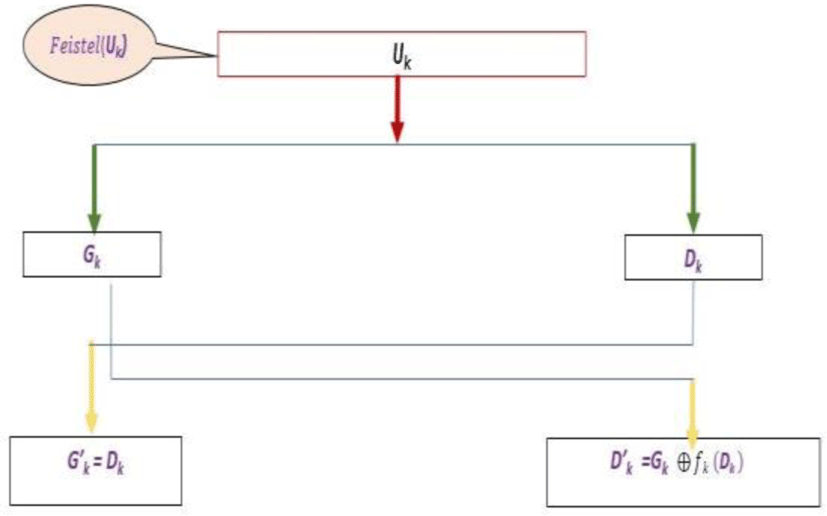Fig. 1. Feistel’s classic round scheme.

This figure could be understood by the following evaluation function. Let t denote the quantity of blocks to be encrypted.

(fi)is a n-bit pseudo-random function.

In the absence of the diffusion, this method stays exposed to differential attacks. As a result, this scheme was expanded to include a new scheme by a bijection construction from purely random functions to produce a new encryption scheme , encapsulating confusion-diffusion. Genetic algorithms are based on the Darwinian evolution of biological populations, whose strongest individuals are the most suitable to survive and reproduce very powerful progeny. These algorithms have surfaced as pre-selected evaluation optimization tools for an assessment function. They are based on the following genetic operations: The inversion, the crossover the mutation and the insertion. Several tentative implementations for genetic algorithms for encrypting color images have surfaced [9-10]. Some use DNA sequences  for image encryption, others have used these genetic algorithms to upgrade some conventional encryption systems .

## II. THE PROPOSED METHOD

Based on chaos, this technique implements one enhanced Feistel lap followed by a genetic crossover. This new color image encryption scheme focuses on six main axes All these measures are shown in a schematic diagram in the following figure.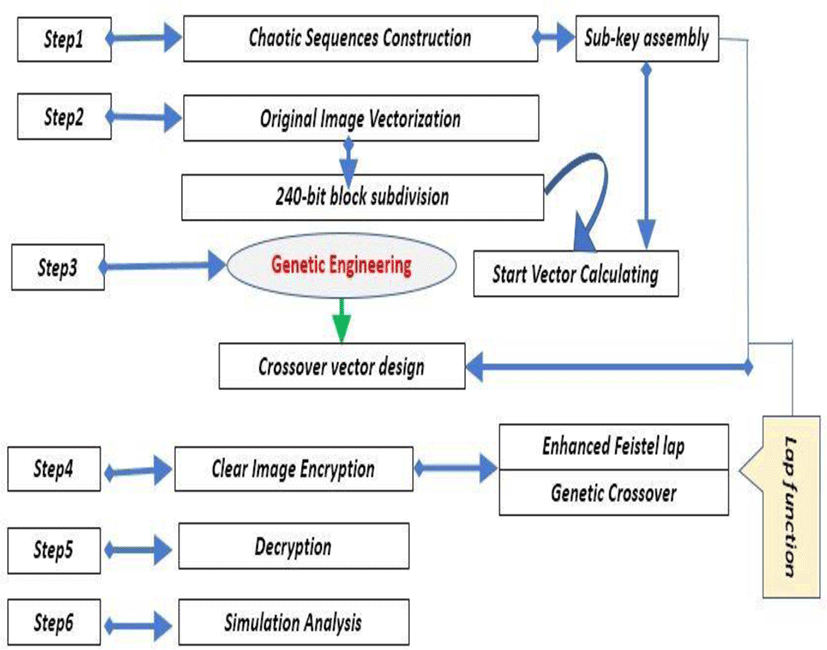Fig. 2. Steps of realization of the algorithm.
2.1. Chaotic Sequences Development

All the encryption parameters necessary for the successful execution of our system are generated from three chaotic maps, the most frequently used in the color image encryption. This choice is due to the simplicity of their exploitation and configuration, as well as their extreme sensitivity to the initial parameters.

2.1.1. The logistics map

The logistic map is a recurrent sequence described by a simple polynomial of second degree defined by the following equation

$\left\{\text{\hspace{0.17em}\hspace{0.17em}\hspace{0.17em}\hspace{0.17em}\hspace{0.17em}\hspace{0.17em}\hspace{0.17em}}\begin{array}{l}{u}_{0}\in \right]\mathbf{0}\mathbf{,}\text{\hspace{0.17em}}\mathbf{5}\text{\hspace{0.17em}\hspace{0.17em}}\mathbf{1}\left[\text{\hspace{0.17em}\hspace{0.17em}\hspace{0.17em}},\text{\hspace{0.17em}\hspace{0.17em}}u\in \left[\mathbf{3}\mathbf{,}\text{\hspace{0.17em}}\mathbf{75}\text{\hspace{0.17em}\hspace{0.17em}\hspace{0.17em}}\mathbf{4}\right]\\ {u}_{n\mathbf{+}\mathbf{1}}=\mu {u}_{n}\left(1-{u}_{n}\right)\end{array}\right\$
(1)

This equation map guarantees that chaos is established to

2.1.2. PWLCM map

It is a real linear sequence by pieces defined by the equation below.

(2)

It is a very simple map to use in color image cryptography. It presents a chaotic aspect for d ∈ [0.51] as control parameters, and w0 ∈ ]01[ as initial conditions.

2.1.3. The skew tent map (SKTM)

The Skew tent map will be redefined as the next equation

(3)

The Skew tent map assures the installation of chaos under the conditions:

2.2. Clear Image Preparation

Before transferred the original image to the encryption surgery center, it must be prepared in anticipation, for this it must include the following activities.

2.2.1. Original image vectoring

After the three (RGB) color channels extraction and their conversion into size vectors (Vr),(Vg),(Vb) (1,nm) each, a concatenation is established to generate a vector X(x1,x2,……,x3nm) of size (1, 3nm)

The resulting vector X(x1,x2,……,x3nm) must be divided into 240 − bit blocks − 30 pixels, therefore its size must be accommodated. Let (l) the new size calculated from the algorithm below.

After, the vector (X) will be transformed into an (TX) vector of size (1,l) by adding 30 – r new chaotic components at the end of the vector (TX), by applying the below algorithm.

2.2.3. 240-bit blocks decomposition

The vector (TX) is converted to binary and then a size (t,240) binary matrix (MC) with (t=l/30). This decomposition can be illustrated by the following figure.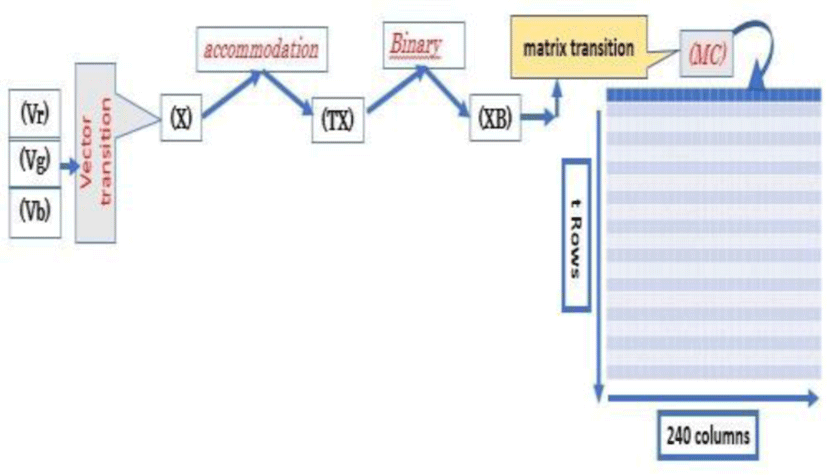Fig. 3. Transition from clear image to matrix (MC).
2.2.4. (IV) Initialization vector design

Ultimately, the (IV) initialization vector of size (1,240) is provided by the next algorithm.

To surpass the uniform image problem (Black, White …) the vector (IV) will be combined with the chaotic vector (HT) specified by the following algorithm.

The blending of the two vectors is performed by the next algorithm.

This vector has the mission to only modify the value of the first block and start the diffusion confusion process.

(NB): In the absence of such an initialization vector, it is very difficult to follow the encryption scheme correctly.

2.3. Encryption Parameter Setting Architecture
2.3.1. Feistel’s first round functions construction

Each MC(k:) block of order (k) will be subdivided into four identical 60 – bit blocks [MG(k:)GM(k:)MD(K:)DM(k:)] and projected to a first enhanced feistel loop described by the following figure.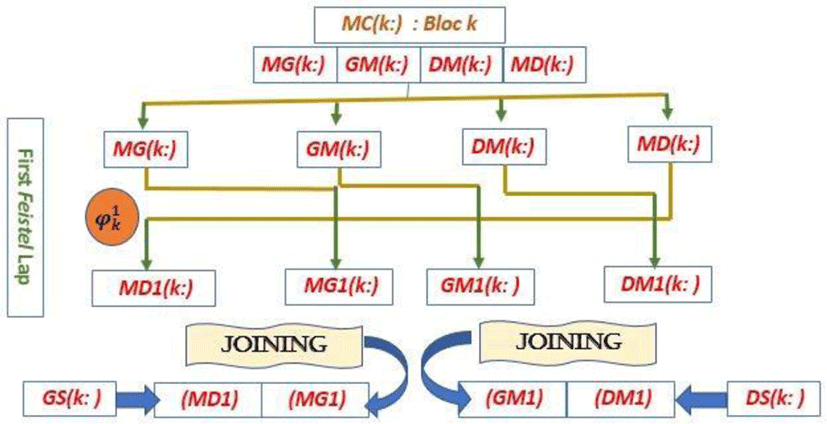Fig. 4. First improved Feistel lap.

This pattern can be analytically expressed by the following mathematical statement.

• hk : Chaotic permutation

• gk : Chaotic displacement

• fk : Chaotic confusion

2.3.1.1. Feistel function lap design
2.3.1.1.1. Permutation scheme (hk) building

Initially, a descending sort on the first 60 values of the logistics sequence generates a permutation (PR) in G60. secondly, a chaotic vector (DP) is constructed in parallel to serve as building the permutation matrix (MP).

The first line of the permutation matrix (MP) is the permutation (PR); while line (i2) is the displacement of line (i−1) of step DP(i). This construction is described by the following algorithm.

Therefore, the application of the permutation hk on the block (MD(k:)) given by the next algorithm.

2.3.1.2. Function scheme gk

gk: is a chaotic offset applied to the 60 bits of the MG(k:) block. This offset is performed by the chaotic vector (DD) resulting by applying the next algorithm.

The analytical expression of such a displacement is illustrated by the following algorithm.

2.3.1.3. Function scheme fk

The matrix (MS) is the passage of the vector (HT) resulting by applying the algorithm4 in matrix of size (t,60). As a result, the confusion function is given by the following algorithm.

2.3.2. Analytical expression of the function ${\phi }_{k}^{1}$

The transformer of first-round diagram block (MC(k:)) given by the algorithm below.

We affirm that the function for the first round is a bijection, its reciprocal is given by the following equation.

2.3.3. Crossover matrix design

A genetic crossover is a pseudo-random function applied to two genes of the same size to form another gene of double size. In our approach, it is a chaotic crossing between two 120-bit vectors to generate a 240-bit block. Firstly, for each block (k) two chaotic vectors (HR) and (RH)are generated by the following algorithm.

So

The crossing function in our system is defined by the following mathematical formula.

The vector (Q) obtained by the following formula.

Example: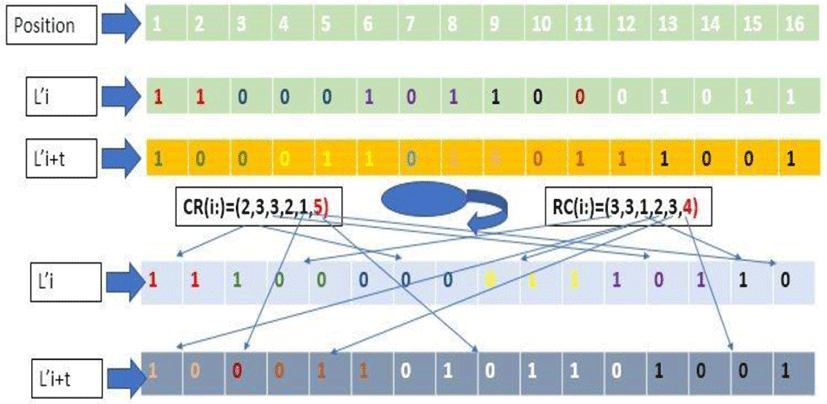Fig. 5. Crossing of two individuals.

The genetic crossing function (Cr) defined by the equation 7 is a bijection. Indeed, we have the block.

$Q=\left({Q}_{1}\right)\left({Q}_{2}\right)\left({Q}_{3}\right)\left({Q}_{4}\right)\dots \dots \dots ..\left({Q}_{24}\right)$
2.4. Clear Image Encryption

Let’s assume (∅) the clear image (MC) encryption function, we have

Therefore

The encryption process can be illustrated by the following diagram.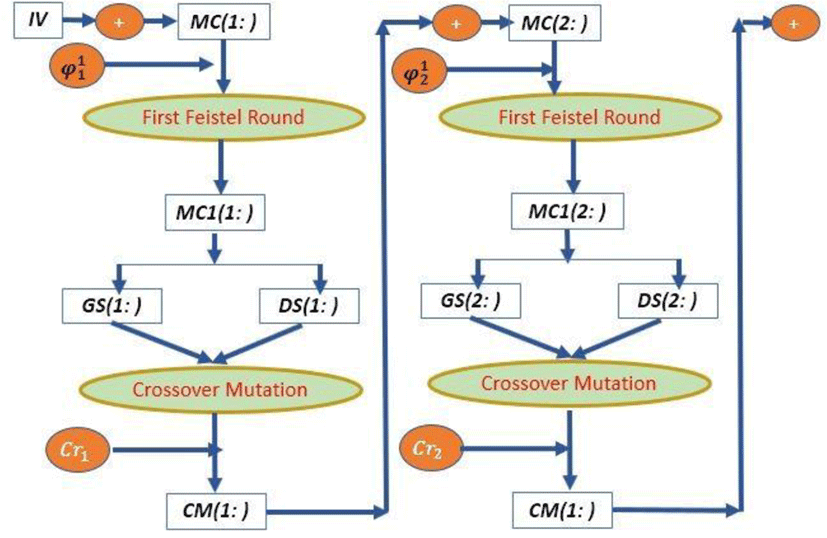Fig. 6. Color image encryption.
2.4.1. Cryptographic function mathematical expression

This new color image encryption technique follows the steps of the algorithm below.

1. Clear image vectoring

2. Adapt the size of image vector

3. Split into t 240 – bit block

4. Extract the initialization vector

5. Do k=1

6. Confusion with the first block

7. Applying the rotation function ${\phi }_{k}^{1}$ the block

8. Perform a genetic crossover on the two output blocks to get the block (IS)

9. Do (IV) = (IS)

10. Do k = k+1

11. If k ≤ t then do MC(k−1:)=MC(k: )

13. If k > t then restore the encrypted image

2.5. Encrypted Image Decryption

Our approach is a symmetrical chaos-based encryption system, so the secret encryption key is also the decryption key. After decomposing the encrypted image into 240-bit blocks and regenerating all encryption parameters, the decryption process starts with the last block by applying the inverse turn function to the second block, then the initialization vector is recalculated to retrieve the first block and restore the original image.

2.6. Example and Simulations

A good system crypto must face all known attacks. For each statistical constant, 150 images are randomly selected according to a chaotic vector from a database of color images of different sizes and formats are tested by our algorithm, and a detailed statistical study has been developed.

2.6.1. Key space

If the precision of the computing is 10 decimal digits, then the size of the encryption key in our approach is 1060 ≈ 2180 ≫ 2100 which is more than enough to protect our method from brutal attacks.

2.6.2. Secret key’s sensitivity analysis

The high sensitivity of the encryption keys used in our system indicates that a very slight degradation of the encryption key automatically leads to an image that is so different from the original image. This confirmation can be viewed below the scheme in the figure12.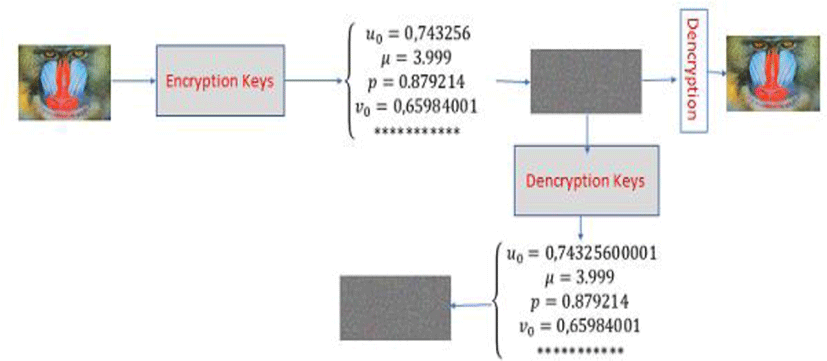Fig. 7. Secret key’s sensitivity

We note that a 10−12 change in a single encryption parameter of this technology is incapable of restoring the clear image by the same decryption process.

2.6.3. Entropy analysis

Entropy is the measure of the disorder diffused by a source without memory. The entropy is therefore maximal for a source whose symbols are all equiproable or presenting a flat histogram. The entropy is for an (MC) image of size (n,m), we pose (t=nm), So

$H\left(MC\right)=\frac{1}{t}\sum _{i=1}^{t}-p\left(i\right){\mathrm{log}}_{2}\left(p\left(i\right)\right)$
(14)

The entropy values on the 150 images tested by our method are represented graphically by the following figure.Fig. 8. Entropy of 150 images of the same size

The entropy values of the images encrypted by our algorithm are around 8, it is the maximum value for a color image encoded on 8 bits. It confirms the uniformity of the histograms. This proves that this approach is safe from entropy attack.

2.6.3.1. Entropy statistical analysis
2.6.3.1.1. Position parameter analysis

The values derived from the entropy by applying our approach to over 150 images in our image database, constitute a statistical series with position, dispersion and concentration parameters have been recalculated to verify the safety of our approach.

(15)
Table 1. Position Parameter
Average Max Min Q1 Q2 Q3
7,999480662 7,999993872 7,999004283 7,99921863 7,99946043 7,999737

The moustache box of the entorpy is illustrated in the diagram in Figure below.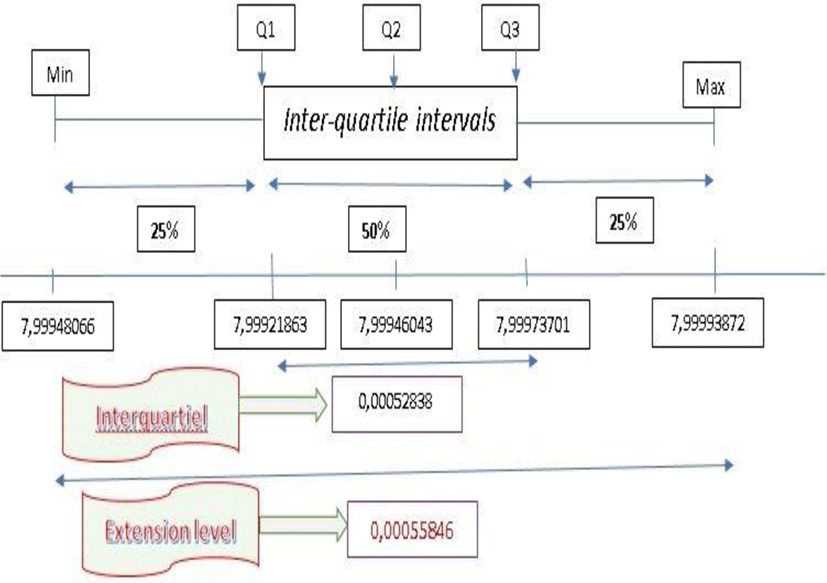Fig. 9. Entropy moustache box.
2.6.3.1.2. Asymmetry coefficient

The Yule coefficient measures the asymmetry of the frequency curve of a statistical series. It is explained by the next equation.

(16)

Under these conditions, Yule has demonstrated that

(17)

In our entropy study, we found

We note that s0.

We can say that the frequency curve is symmetrical.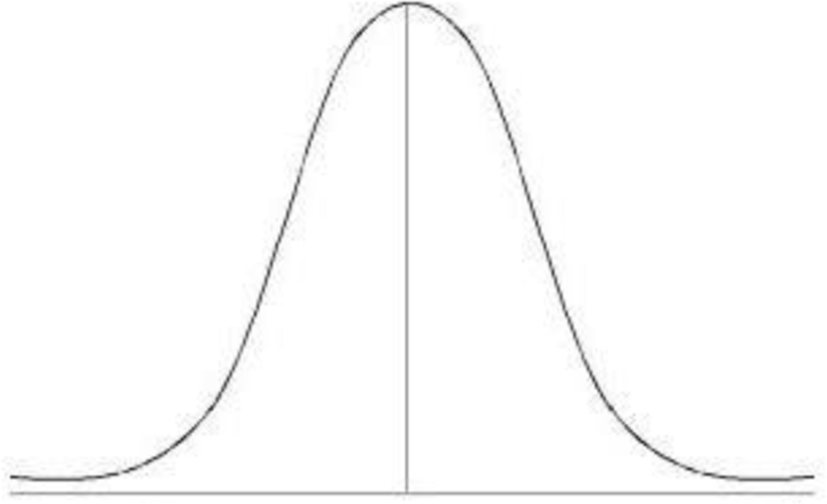Fig. 10. Example of a symmetrical curve following a normal distribution (s≈0).
2.6.3.1.3. Applatissment coefficient

Flattening is judged by reference to the normal distribution density curve model. We will say that the frequency curve is more or less flattened than the normal distribution model.

The coefficient for quantitatively measuring flattening is called the (Kurtosis). Pearson proposed the following coefficient:

(18)

Under these conditions, Pearson has demonstrated that

(19)

In our entropy study, we found

We note that β2 ≈ 3.

We can say that our distribution is a normal distribution.

2.6.3.2. Correlation analysis

Correlation is a technique that compares two images to estimate the displacement of pixels in one image relative to another reference image. Adjacent pixels of a standard image of a clear image have a strong correlation. A good crypto image system must remove such correlation in order to avoid any statistical attack. The correlation expression is defined by equation below.

(20)
2.6.3.2.1. Horizontal correlation

Simulations performed on 100 identical-sized color images choose from a wide database of images of various sizes, formats and correlated values are represented graphically by the next figure.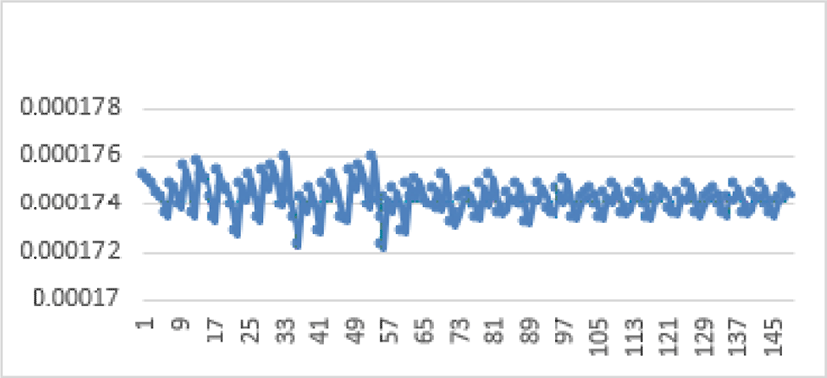Fig. 11. Entropy of 100 images of the same size.
2.6.3.2.2. Vertical correlation

Simulations made on 150 images of the database gave the vertical correlation scores are displayed in Figure below.

Figure 12 shows that the vertical correlation values of the encrypted images are close to zero. This ensures high security against correlation attacks.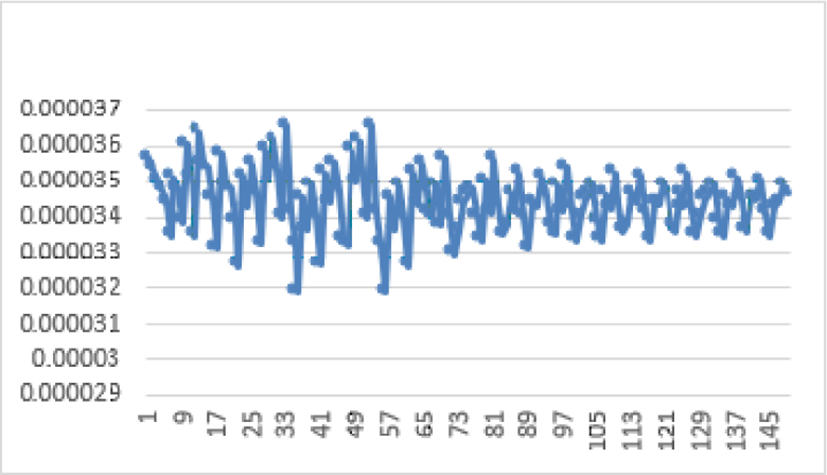Fig. 12. Vertical correlation of 70 images of the varying sizes.
2.6.3.2.3. Diagonal correlation

Simulations made on 150 images of the database gave the diagonal correlation scores are displayed in Figure 13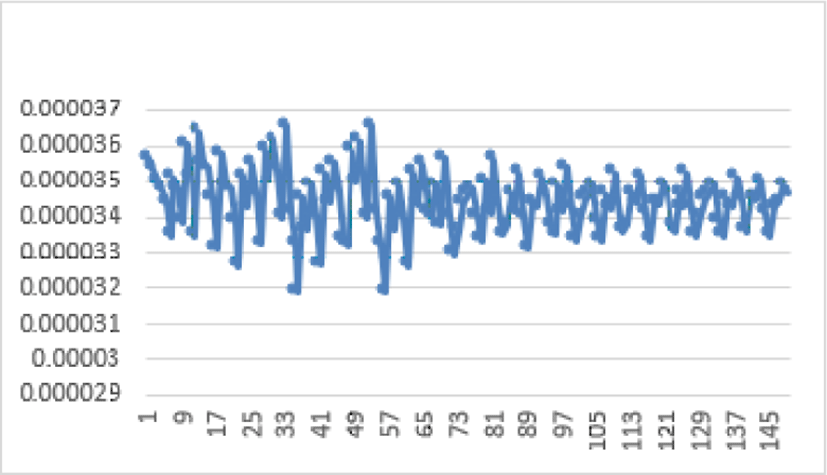Fig. 13. Diagonal correlation of 100 images of the varying sizes.

Figure 11 shows that the diagonal correlation values of the encrypted images are close to zero. This ensures high security against correlation attacks.

2.6.3.3. Differential analysis

Let be two encrypted images, whose corresponding free-to-air images differ by only one pixel, from (C1) and (C2), respectively. The expressions of these two statistical constants (NPCR)and (UACI) are given by equations 12 and 13, for an image size (n,m).

The NPCR mathematical analysis of an image is given by the equation below.

$NPCR=\left(\frac{1}{nm}\sum _{i,j=1}^{nm}D\left(i,j\right)\right)*100$
(21)

With

The UACI mathematicals analysis of an image is given by the equation 36

$UACI=\left(\frac{1}{nm}\sum _{i,j=1}^{nm}Abs\left({C}_{1}\left(i,j\right)-{C}_{2}\left(i,j\right)\right)\right)*100$
(22)

The study of the 150 selected images revealed the following diagram.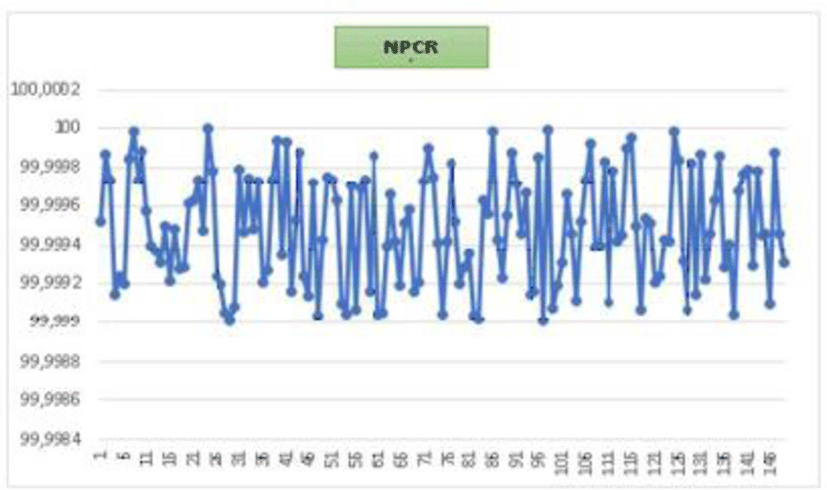Fig. 14. NPCR of 150 images of the varying sizes.

All detected values are inside the confidence interval [99,63 99,95]. These values are largely sufficient to affirm that our crypto system is protected from known differential attacks.

The study of the 150 selected images revealed the following diagram.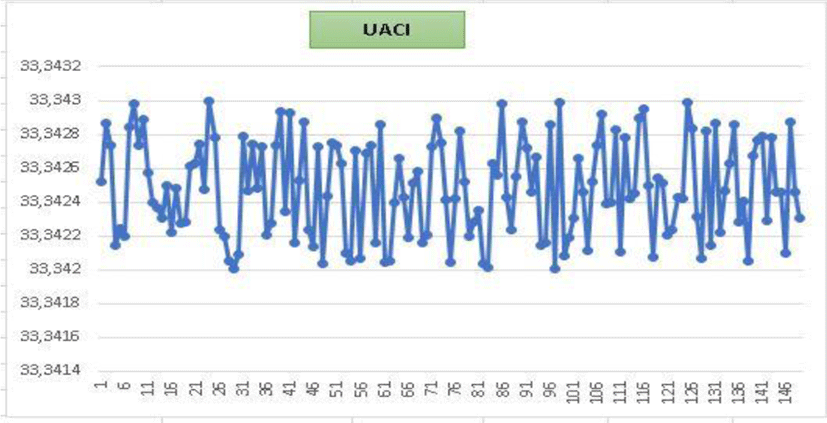Fig. 15. UACI of 150 images of the varying sizes.

All detected values are inside the confidence interval [33,34 33,35]. These values are largely sufficient to affirm that our crypto system is protected from known differential attacks.

2.6.3.4. Avalanche effect

The avalanche effect is a required property in virtually all cryptographic hash functions and block coding algorithms. It causes progressively more important changes as the data is propagating in the structure of the algorithm. Therefore, by perturbing a single bit at the input, we can obtain a very different output, (about 1 bit our of 2 changed) explaining the name of this phenomenon. The avalanche effect makes it more difficult to reverse the function due to its chaotic properties (if well designed).

This constant determines the avalanche impact of the cryptographic structure in place. It is approximated by the next equation.

(23)

Figure below depicts the evaluation of the AE score for 150 images examined by our approach.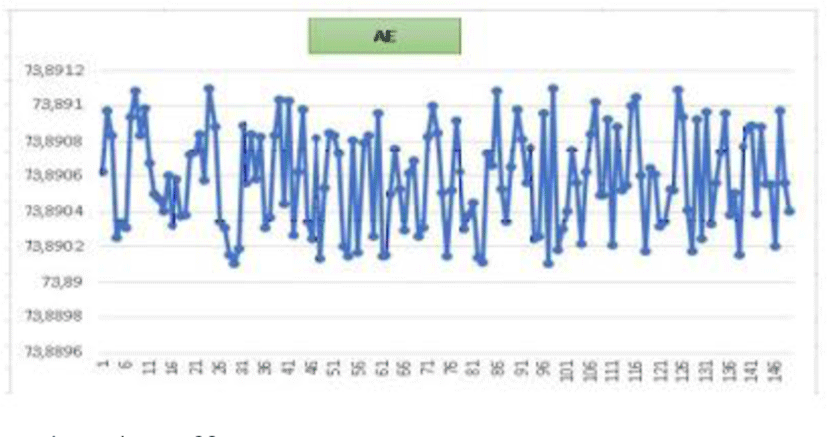Fig. 16. Avalanche effect.

All values returned from the AE by our method are all in the range of residual values [73.96 74.02]. This guarantees that a one-bit change in the clear image will be reflected by a change of at least 78% of the encrypted image’s bits.

2.6.3.5. Signal-To-Peak noise ratio (PSNR)
2.6.3.5.1 MSE

The image quality estimation to be based on the pixel change was obtained by processing the PSNR values and the MSE. These are the error metrics used to compare the image and the cipher image.

Mean Square Error MSE: This is the cumulative square deviation between the original image and the additional noise image. When the MSE level is reduced, the error is reduced.

This constant measure the distance between the pixels of the clear image and the encrypted image. It is calculated by the next equation.

$MSE=\sum _{i,j}{\left(P\left(i,j\right)-C\left(i,j\right)}^{2}$

(P(i,j)) : pixel of the clear image

(C(i,j)) : pixel of the cypher image

2.6.3.5.2 PSNR

The signal-to-peak noise ratio, often abbreviated PSNR, is a engineering term for the ratio between a signal’s maximum possible power and the power of distorted noise that affects the precision of its display. Since many signals have a very large dynamic range, the PSNR is generally stated in terms of the logarithmic decibel scale. The PSNR mathematical analysis of an image is given by the next equation.

(25)

For RGB color images, the definition of PSNR is the same except that the MSE is the sum of all square value changes. In the alternative, for color images, the image is transcoded into a separate color space and the PSNR is displayed for each channel in that color space. The acceptable PSNR values are the real numbers in the domain (5,10). Simulations made on over 150 images of various magnitudes and formats returned the same results as depicted in the figure 17.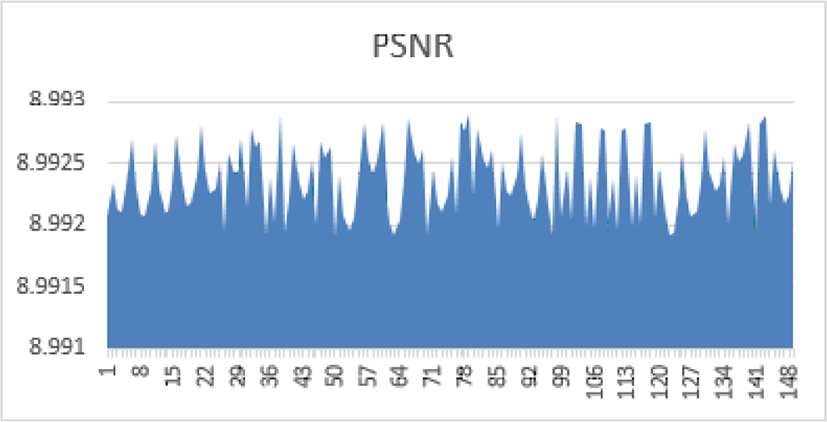Fig. 17. PSNR of 150 images of the varying sizes.

All values returned from the PSNR by our method are all in the range of residual values [8,99 8,993]. A statistical study of the dispersions of the PSNR values of the 150 images analyzed by our algorithm reveals the scores presented in the following Table 1:

Table 1. Position Parameter
Constant Value
Average 8,992375198
Max 8,992889912
Min 8,99190426
Q1 8,99208424
Q2 8,9923513
Average 8,992375198
Q3 8,99260954
s -0,001679040
β2 2,980021

This table ensures that there is a low dispersion and a high concentration of values in a length interval of 0.001. Moreover, the value of s close to 0 indicates that there is a symmetry in this dispersion and the value of β2close to 3 shows that our dispersion follows a normal distribution. More than 50% of the values achieved are within a longitudinal range of less than 0.0006.

2.6.3.6. Speed analysis

Assuming that the traditional DES and AES encryption algorithms operate in ECB mode, they are vulnerable to statistical attacks and selected plain text attacks. In addition, these two systems require no linking on clear and encrypted blocks and are consequently deficient in the face of differential attacks. In this sense, we will compare the time complexity for reference images with these two crypto systems. In addition to safety parameters, runtime is an important factor in evaluating image encryption system performance. To approve and document the quality of our methodology in a timely fashion. And finally, thanks to these properties, we have selected the "Lena" grayscale image with three different sizes (256×256) (512×512) and (1024×1024). The results are presented in the table below.

Table 2. Execution time (in second).
Size Our Method DES AES Classic Hill Hill 
256×256 0,097 0,639 0,568 0,192 0,081
512×512 0,172 7,449 0,354 0,214 0,201
1024×1024 0,201 29,112 1,152 0,921 0,852

We compare our results with the two classical algorithms AES and DES, Classic Hill and Improvement Hill, we can affirm that the time of execution is reasonable. The test was performed on other images of different sizes, and we obtained acceptable scores. This is due to the low algorithm complexity of the implemented algorithms in our strategy.

2.6.3.7 Math security

The large size of our encryption key ensures that the system is protected against any brute force attack. At the same time, the randomness of the genetic operator and the functions of Feistel’s trick make it difficult to unlock the encryption system applied to a given block, increasing the difficulty of the statistical attack. In addition, the high sensitivity to the initial parameters of our three chaotic maps, and the statistical constants calculated in simulation make it difficult to reconstruct the encryption key.

## III. CONCLUSION

Taking security as its primary objective, this document develops a new encryption framework for color images of arbitrary size. Based on chaos, this technique relies on 24-bit blocks by applying an improved Feistel round accompanied by genetic crossover, followed by chaining to install protection against any known attack. Simulations performed on more than 150 randomly selected images from a large database of color images of different sizes and formats confirm the robustness of our system.

## REFERENCES

.

C. E Shanon, Communication theory of security systems, Bell syst Tech J., pp 656-715, 1949.

A.Jarjar, Improvement of hill’s classical method in image cryptography, International Journal of Statistics and Applied Mathematics, vol. 2, no. 3, Part A, 2017..

Hraoui S., Gmira F., Jarar A. O., Satori K. and Saaidi A., Benchmarking AES and chaos based logistic map for image encryption, in Proceeding ofInternational Conference Computer Systems and Applications (AICCSA), 2013..

G. Zhang, Q. Liu, "A novel image encryption method based on total shuffling scheme", Opt. Commun., pp. 284-2775, 2011..

Xiao Feng, Xiaolin Tian and Shaowe iXia, "An Improved Image Scrambling Algorithm Based On Magic Cube Rotation and Chaotic Sequences", in Proceeding of IEEE 4th International Congress on Image and Signal Processing, pp. 1021-1024, 2011..

Chang'e Dong, Color image encryption using one-time keys and coupled chaotic systems, Signal Processing: image Communication, vol. 29, no. 5, pp. 628-640, 2014..

Xing-Yuan Wanga, Sheng-Xian Gua and Ying-Qian Zhangab, “Novel image encryption algorithm based on cycle shift and chaotic system,” Signal Processing: Image Communication. vol. 68, pp. 126-134, 2015..

G. Zhang and Q. Liu, “A novel image encryption method based on total shuffling scheme”, Opt. Commun., pp. 2775-2780,2011..

Tomoyasu Suzaki Kazuhiko Minematsu, “Improving the Generalized Feistel,” in Proceeding of International Workshop on Fast Software Encryption FSE 2010, pp. 19-39, 2010..

Abdellatif JarJar, “Improvement of Feistel method and the new encryption scheme,” Optik, vol. 157, pp. 1319-1324, 2018..

Jacques Patarin, “Security of Random Feistel Schemes with 5 or More Rounds,” in Proceeding of Annual International CryptologyConference CRYPTO 2004: Advances in Cryptology – CRYPTO, pp. 106-122, 2004.## Author

ABDELLATIF JARAJARis Cryptography Researcher. His major concern is a proof mathematics and he is a member of Moulay Rachid Hight School, Taza, in Morroco.